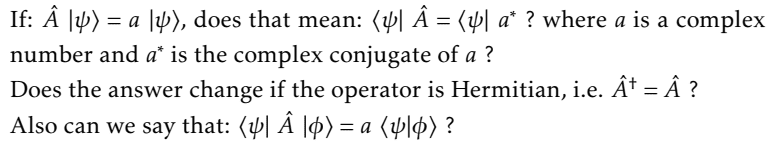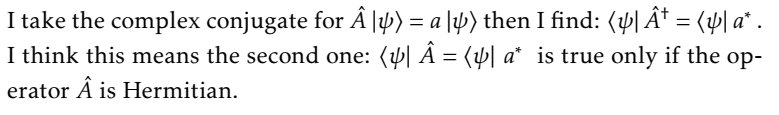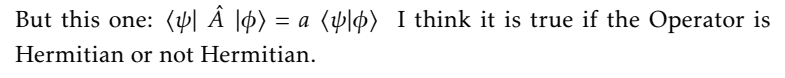# Operator acts on a ket and a bra using Dirac Notation

Thread moved from the technical forums to the schoolwork forums
Summary:: Operator acts on a ket and a bra using Dirac NotationPeroK
Homework Helper
Gold Member
2020 Award
We should treat this as a homework problem. Can you make an attempt at answering it?PeroK
Homework Helper
Gold Member
2020 Award
Looks good. Can you say anything more if ##\hat A## is Hermitian?

PeroK
Homework Helper
Gold Member
2020 Award
Can you prove it?

Nugatory
Mentor
Summary:: Operator acts on a ket and a bra using Dirac Notation

Please take a few minutes to learn how to use this site's Latex feature.... There's a guide in the help section at https://www.physicsforums.com/help/latexhelp/

•Viona
Looks good. Can you say anything more if ##\hat A## is Hermitian?
If A is Hermitian then the eigenvalue (a) is a real number.

•PeroK
Can you prove it?
I am not sure if this is true or not.

PeroK
Homework Helper
Gold Member
2020 Award
I am not sure if this is true or not.
I don't think it is true in general for any operator. Certainly for Hermitian operators and also for normal operators (these are operators that commute with their Hermitian conjugate), but not in general.

•Viona
PeroK
Homework Helper
Gold Member
2020 Award
Switching to normal linear algebra notation. If ##A## commutes with ##A^{\dagger}## and ##v## is an eigenvector of ##A## with eigenvalue ##a##, then:
$$A(A^{\dagger}v) = A^{\dagger}(Av) = A^{\dagger}(av) = a(A^{\dagger}v)$$ and we see that ##A^{\dagger}v## is an eigenvector of ##A## with eigenvalue ##a##. Which means that ##A## and ##A^{\dagger}## share eigenspaces.

And it's easy to show that the eigenvalues are complex conjugates.

You can use that to prove the identity in your question, but I think you need that condition.

•docnet and Viona
I don't think it is true in general for any operator. Certainly for Hermitian operators and also for normal operators (these are operators that commute with their Hermitian conjugate), but not in general.
Can we say that if the operator is Hermitian then: <ψ| A |Φ> =<Φ| A |ψ>*= a <Φ | ψ>* = a <ψ | Φ> ?

•PeroK
PeroK
Homework Helper
Gold Member
2020 Award
Yes, and if we assume that ##A## and ##A^{\dagger}## share eigenvectors with cc eigenvalues, then: $$\langle \psi |A| \phi \rangle = \langle \phi |A^{\dagger}| \psi \rangle^* = \langle \phi |a^*| \psi \rangle^* = a\langle \psi |\phi \rangle$$ So, that's slightly more general than Hermitian, with ##A## normal and non-degenerate.

•Viona
Yes, and if we assume that ##A## and ##A^{\dagger}## share eigenvectors with cc eigenvalues, then: $$\langle \psi |A| \phi \rangle = \langle \phi |A^{\dagger}| \psi \rangle^* = \langle \phi |a^*| \psi \rangle^* = a\langle \psi |\phi \rangle$$ So, that's slightly more general than Hermitian, with ##A## normal and non-degenerate.
It is clear now. It seems to me that I need to educate myself and study more in linear algebra. Thank you for your help!!!

PeroK
PS ultimately it's simply this equality you need: $$A^{\dagger}| \psi \rangle = a^*| \psi \rangle$$ And that holds for Hermitian operators, some other operators, but not all operators.